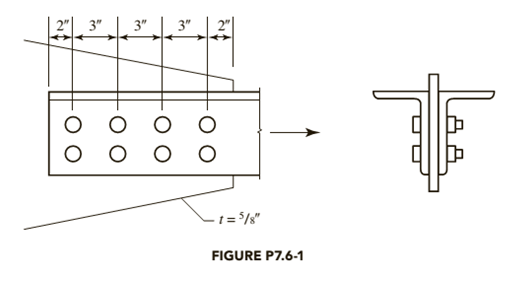# A double-angle Shape, 2 L 6 × 6 × 5 8 , is connected to a 5 8 -inch gusset plate as shown in Figure P7.6-l. Determine the maximum total service lead that can be applied if the ratio of dead load to live load is 8.5. The bolts are 7 8 -inch-diameter, Group A bearing-type bolts. A572 Grade 50 steel is used for the angle, and A36 steel is used for the gusset plate. a . Use LRFD. b . Use ASD.### Steel Design (Activate Learning wi...

6th Edition
Segui + 1 other
Publisher: Cengage Learning
ISBN: 9781337094740

#### Solutions

Chapter
Section### Steel Design (Activate Learning wi...

6th Edition
Segui + 1 other
Publisher: Cengage Learning
ISBN: 9781337094740
Chapter 7, Problem 7.6.1P
Textbook Problem
13 views

## A double-angle Shape, 2 L 6 × 6 × 5 8 , is connected to a 5 8 -inch gusset plate as shown in Figure P7.6-l. Determine the maximum total service lead that can be applied if the ratio of dead load to live load is 8.5. The bolts are 7 8 -inch-diameter, Group A bearing-type bolts. A572 Grade 50 steel is used for the angle, and A36 steel is used for the gusset plate.a. Use LRFD.b. Use ASD.To determine

(a)

The maximum total load by using Load and Resistance Factor Design (LRFD) method.

### Explanation of Solution

Given:

Double angle section is 2L6×6×58.

Thickness of the gusset plate is 58inch.

Diameter of the bolts is 78inch.

Steel used for angle is A572, Grade 50.

Steel Used for gusset plate is A36.

Calculation:

The properties for 2L6×6×58 section from the AISC steel table are as follows:

The gross area is 7.13inch2.

The distance from the plane of connection to the centroid is 1.72inch.

The properties for A36 steel from the AISC steel table are as follows:

The ultimate tensile stress is 58ksi.

Write the expression for cross-sectional area of the bolt.

Ab=π4d2     ...... (I)

Here, cross-sectional area of the bolt is Ab and diameter of the bolt is d.

Substitute 78inch for d in Equation (I).

Ab=π4(78inch)2=0.601inch2

Write the expression for nominal shear capacity one bolt.

Rnv=FnvAb     ...... (II)

Here, nominal shear stress of one bolt is Rnv and nominal shear stress of the bolt material is Fnv.

Substitute 0.601inch2 for Ab and 54ksi for Fnv in Equation (II).

Rnv=(54ksi)(0.601inch2)×2=64.94kips

Write the expression to calculate the strength.

S=n×Rnv     ...... (III)

Here, number of bolts is n and strength is S.

Substitute 8 for n and 64.94kips for Rnv in Equation (III).

S=8×64.94kips=519.52kips

Write the expression to calculate the nominal bearing strength of a bolt Rn.

Rn=1.2lctFu     ...... (IV)

Here, nominal bearing strength is Rn, thickness of the gusset plate is t, ultimate tensile stress is Fu, and the distance between the edge of the adjacent hole to the edge of the bolt hole is lc.

With the upper limit of nominal bearing strength of the bolt,

Rn=2.4dtFu

Write the expression to calculate the value of lc for the edge bolts.

lc=leh2     ...... (V)

Here, height of the bolt is h.

Write the expression to calculate the value of lc for inner bolts.

lc=sh     ...... (VI)

Here, spacing between the bolts is s.

Write the expression to calculate the height of the bolt.

h=d+116inch     ...... (VII)

Calculate the value of h.

Substitute 78inch for d Equation (VII).

h=78inch+116inch=1516inch

Calculate the value of lc for edge bolts.

Substitute 2inch for le and 1516inch for h in Equation (V).

lc=2inch(1516inch)2=1.531inch

Calculate the nominal bearing strength for edge bolts.

Substitute 1.531inch for lc, 58inch for t, and 58ksi for Fu in Equation (IV).

Rn=1.2(1.531inch)(58inch)(58ksi)=66.6kips

Calculate the upper limit of nominal bearing strength for edge bolts.

Substitute 78inch for d, 58ksi for Fu, and 58inch for t.

Rn=2.4(78inch)(58inch)(58ksi)=76.13kips

Thus, the minimum value of Rn is considered and is equal to 66.6kips.

Calculate the value of lc for inner bolts.

Substitute 3inch for s and 1516inch for h in Equation (VI).

lc=3inch1516inch=2.063inch

Calculate the nominal bearing strength for inner bolts.

Substitute 2.063inch for lc, 58inch for t, and 58ksi for Fu in Equation (IV).

Rn=1.2(2.063inch)(58inch)(58ksi)=89.74kips

Calculate the upper limit of nominal bearing strength for inner bolts.

Substitute 78inch for d, 58ksi for Fu and 58inch for t.

Rn=2.4(78inch)(58inch)(58ksi)=76.13kips

Thus, the minimum value of Rn is considered and is equal to 76.13kips.

Write the expression to calculate the nominal strength in yielding.

Pn=FyAg

To determine

(b)

The maximum total allowable load by using Allowable Stress Design (ASD) method.

### Still sussing out bartleby?

Check out a sample textbook solution.

See a sample solution

#### The Solution to Your Study Problems

Bartleby provides explanations to thousands of textbook problems written by our experts, many with advanced degrees!

Get Started

Find more solutions based on key concepts
Describe the basic ideas of probability.

Engineering Fundamentals: An Introduction to Engineering (MindTap Course List)

Define a class, subclass, and superclass, and provide three examples of each.

Systems Analysis and Design (Shelly Cashman Series) (MindTap Course List)

What is meant by an organizations technology infrastructure?

Principles of Information Systems (MindTap Course List)

List and describe seven criteria that make information valuable.

Enhanced Discovering Computers 2017 (Shelly Cashman Series) (MindTap Course List)

Does all of the heat produced by an SMA weld stay in the weld? Why or why not?

Welding: Principles and Applications (MindTap Course List)

If your motherboard supports ECC DDR3 memory, can you substitute non-ECC DDR3 memory?

A+ Guide to Hardware (Standalone Book) (MindTap Course List)